integration by trial and error. However, there are some rules to aid us in the determination of the answer. In this section we will discuss four of these rules and how they are used to integrate standard elementary forms. In the rules we will let u and v denote a differentiable function of a variable such as x. We will let C, n, and a denote constants."> Rules for IntegrationCustom SearchRULES FOR INTEGRATION Although integration is the inverse of differentiation and we were given rules for differentiation, we are required to determine the answers in integration by trial and error. However, there are some rules to aid us in the determination of the answer. In this section we will discuss four of these rules and how they are used to integrate standard elementary forms. In the rules we will let u and v denote a differentiable function of a variable such as x. We will let C, n, and a denote constants. Our proofs will involve searching for a function F(x) whose derivative is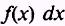.The integral of a differential of a function is the function plus a constant. PROOF: If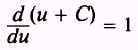then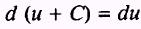and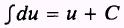EXAMPLE. Evaluate the integral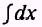SOLUTION: By Rule 1, we haveA constant may be moved across the integral sign. NOTE: A variable may NOT be moved across the integral sign. PROOF: If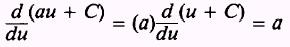thenandEXAMPLE: Evaluate the integralSOLUTION: By Rule 2,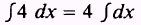and by Rule 1,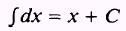therefore,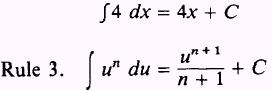The integral of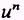du may be obtained by adding 1 to the exponent and then dividing by this new exponent. NOTE: If n is minus 1, this rule is not valid and another method must be used. PROOF.- IfthenEXAMPLE: Evaluate the integral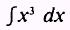SOLUTION: By Rule 3,EXAMPLE: Evaluate the integral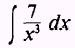SOLUTION: First write the integralas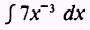Then, by Rule 2,and by Rule 3,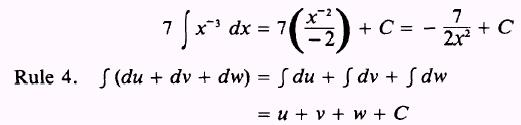The integral of a sum is equal to the sum of the integrals. PROOF: If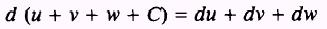then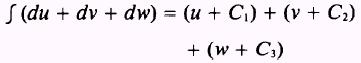such thatwhereEXAMPLE: Evaluate the integral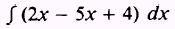SOLUTION: We will not combine 2x and -5x.where C is the sum of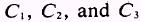. EXAMPLE: Evaluate the integral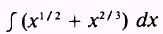SOLUTION:Now we will discuss the evaluation of the constant of integration. If we are to find the equation of a curve whose first derivative is 2 times the independent variable x, we may writeor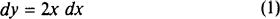We may obtain the desired equation for the curve by integrating the expression for dy; that is, by integrating both sides of equation (1). Ifthen,But, since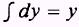and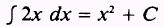then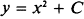We have obtained only a general equation of the curve because a different curve results for each value we assign to C. This is shown in figure 6-7. If we specify that x=0 And y=6 we may obtain a specific value for C and hence a particular curve. Suppose thatthen,or C=6Figure 6-7.-Family of curves. By substituting the value 6 into the general equation, we find that the equation for the particular curve iswhich is curve C of figure 6-7. The values for x and y will determine the value for C and also determine the particular curve of the family of curves. In figure 6-7, curve A has a constant equal to - 4, curve B has a constant equal to 0, and curve C has a constant equal to 6. EXAMPLE: Find the equation of the curve if its first derivative is 6 times the independent variable, y equals 2, and x equals 0. SOLUTION. We may write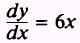orsuch that,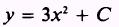Solving for C when x=0 and y=2 We have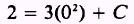or C=2 so that the equation of the curve is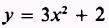Integrated Publishing, Inc. - A (SDVOSB) Service Disabled Veteran Owned Small Business